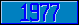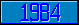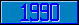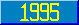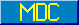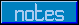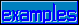## MWAPI - ARC

### drawarc

Introduced in the 1995 ANSI M[UMPS] Windowing Application Programmer's Interface standard.

This metalanguage symbol represents an expression that evaluates to the value "ARC".

### endangle

Introduced in the 1995 ANSI M[UMPS] Windowing Application Programmer's Interface standard.

This metalanguage symbol represents the final angle in the context of a drawing command (ARC or PIE). The angle is measured in degrees. 0 degrees is horizontal, along the positive axis, 90 degrees is vertical along the positive axis.

Introduced in the 1995 ANSI M[UMPS] Windowing Application Programmer's Interface standard.

This metalanguage symbol represents the radius in the context of a drawing command (ARC or PIE). The unit of measure for this value is determined by the value of the UNITS element attribute for the gadget in question.

### startangle

Introduced in the 1995 ANSI M[UMPS] Windowing Application Programmer's Interface standard.

This metalanguage symbol represents the initial angle in the context of a drawing command (ARC or PIE). The angle is measured in degrees. 0 degrees is horizontal along the positive axis, 90 degrees is vertical along the positive axis.